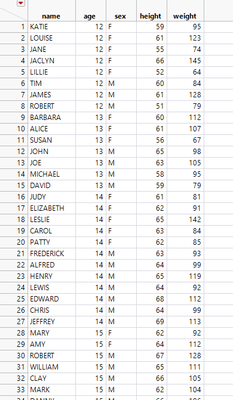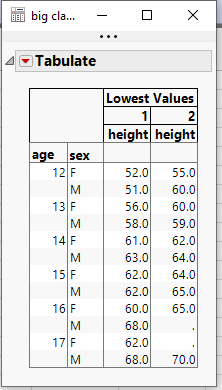Choose Language Hide Translation Bar
Highlighted

## How to get lowest two row values in grouped data ?

Hi,

Lets say we have data like this and want to get lowest two row values.

Group by Age and Sex, get the two lowest height values.

How can we do this in JSL? ThanksHighlighted

## Re: How to get lowest two row values in grouped data ?

Here is one way to do it``````Names Default To Here( 1 );
dt = Open( "\$SAMPLE_DATA/big class.jmp" );

dtSum = dt << Summary(
private,
Group( :age, :sex, :height ),
Freq( "None" ),
Weight( "None" ),
Link to original data table( 0 )
);

dtSum << delete columns( "N Rows" );

dtSum << New Column( "Lowest Values",
ordinal,
formula(
If(
Row() == 1, x = 1,
:age != Lag( :age ) | :sex != Lag( :sex ), x = 1,
x
++);
x;
)
);

dtSum:Lowest Values << delete formula;

dtSum << select where( :Lowest Values > 2 );
dtSum << delete rows;

dtSum << Tabulate(
Change Item Label( Statistics( Mean, " " ) ),
Show Control Panel( 0 ),